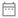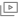Back to Plus

Course on Engineering Mathematics

Aman Raj

1k followers

In this course, Aman Raj will provide in-depth knowledge of Engineering Mathematics. The course will cover all concepts from Complete Course on Engineering Aptitude etc. and would be helpful for aspirants preparing for ESE - GS. Learners at any stage of their preparation will be benefited from the course. The course will be covered in Hindi and the notes will be provided in English. The course includes 38 sessions of 90 minutes each. All doubts related to the topic will be clarified during the doubt clearing sessions in the course.
Course schedule
Jul
22
Linear Algebra - Basics
Lesson 13:30 PM
Jul
24
Linear Algebra - Rank of Matrix
Lesson 23:30 PM
Jul
25
Linear Algebra - System of Linear equation
Lesson 33:30 PM
Jul
26
Doubt Clearing Session
Lesson 42:30 PM
Jul
27
Weekly Quiz
Lesson 59:30 AM
Jul
27
Calculus- Introduction
Lesson 62:30 PM
Jul
28
Calculus - Limit, Continuity & Differentiability
Lesson 73:16 PM
Jul
29
Calculus - Maxima, Minima
Lesson 82:30 PM
Jul
30
Calculus-Taylor's Series
Lesson 92:30 PM
Jul
31
Calculus- Definite & Indefinite Integration
Lesson 102:00 PM
Jul
31
Doubt Clearing Session
Lesson 114:00 PM
Aug
01
Calculus - Application of Integrals
Lesson 122:00 PM
Aug
01
Calculus - Multiple Integrals & Gamma Function
Lesson 134:00 PM
Aug
02
Weekly Quiz
Lesson 141:00 PM
Aug
02
Vector Calculus
Lesson 152:00 PM
Aug
02
Vector Calculus - Jacobian - Change of Variables
Lesson 164:00 PM
Aug
03
Vector Integration
Lesson 172:00 PM
Aug
03
Doubt Clearing Session
Lesson 184:00 PM
Aug
04
Differential Equation
Lesson 192:00 PM
Aug
04
Differential Equation-Linear Differential Equation - Non Homogeneous LDE
Lesson 204:30 PM
Aug
05
Weekly Quiz
Lesson 219:30 AM
Aug
05
Differential Equation - P. D. E
Lesson 222:00 PM
Aug
05
Doubt Clearing Session
Lesson 234:00 PM
Aug
06
Laplace Transforms
Lesson 249:00 AM
Aug
06
Complex Variables
Lesson 252:00 PM
Aug
06
Complex Functions
Lesson 264:00 PM
Aug
07
Doubt Clearing Session
Lesson 279:00 AM
Aug
07
Complex Variables - CR Equations
Lesson 282:00 PM
Aug
07
Complex Variables - Cauchy's Theorm
Lesson 294:00 PM
Aug
08
Residue Theorem
Lesson 302:00 PM
Aug
08
Introduction to Probability
Lesson 314:30 PM
Aug
09
Weekly Quiz
Lesson 322:30 AM
Aug
09
Conditional Probability
Lesson 332:00 PM
Aug
09
Probability - statistics & Distribution
Lesson 344:45 PM
Aug
10
Numerical Method
Lesson 352:30 AM
Aug
10
Doubt Clearing Session
Lesson 362:00 PM
Aug
16
Numerical Method
Lesson 372:30 AM
Aug
16
Probability on Baye's Theorem
Lesson 381:30 PM
Aug
16
Probability on Statistics
Lesson 394:00 PM
Aug
17
Probability on Distributions
Lesson 402:30 AM
Aug
17
Miscellaneous Topics
Lesson 414:30 PM
Aug
25
Mega Quiz
Lesson 422:30 AM
Aug
25
Doubt Clearing Session
Lesson 438:30 AMCourse will be taught in Hindi22nd July to 25th August 201938 lessons, 5 quizzes